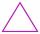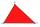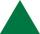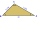# Triangle + inequalities - math problems

#### Number of problems found: 10

• 3-bracket 2May be the smallest angle in the triangle greater than 70°?
• 3-bracketMay be the largest angle in the triangle less than 20°?
• TriangleFor how many integer values of x can 6, 19 and x be the lengths of the sides of triangle?
• 3-bracket 3Two angles in a triangle are 90° and 60°. Has triangle at least two equal sides?
• QuizQAn isosceles triangle has two sides of length 7 km and 39 km. How long is a third side?
• R triangleCalculate the right triangle area whose longer leg is 6 dm shorter than the hypotenuse and 3 dm longer than the shorter leg.
• Right triangle AlefThe obvod of a right triangle is 84 cm, and the hypotenuse is 37 cm long. Determine the lengths of the legs.
• The perimeterThe triangle has one side 5 cm long and the another 11 cm long. What can be the smallest and what is the largest perimeter?
• TrianglesIvo wants to draw all the triangles whose two sides of which have a length of 4 cm and 9 cm, and the length of the third side is expressed in whole centimeters. How many triangles does he have?
• Widescreen monitorComputer businesses hit by a wave of widescreen monitors and televisions. Calculate the area of ​​the LCD monitor with a diagonal size 20 inches at ratio 4:3 and then 16:9 aspect ratio. Is buying widescreen monitors with the same diagonal more advantageou

We apologize, but in this category are not a lot of examples.
Do you have an interesting mathematical word problem that you can't solve it? Submit a math problem, and we can try to solve it.

We will send a solution to your e-mail address. Solved examples are also published here. Please enter the e-mail correctly and check whether you don't have a full mailbox.

Please do not submit problems from current active competitions such as Mathematical Olympiad, correspondence seminars etc...

See also our trigonometric triangle calculator. Triangle Problems. Inequalities - math problems.